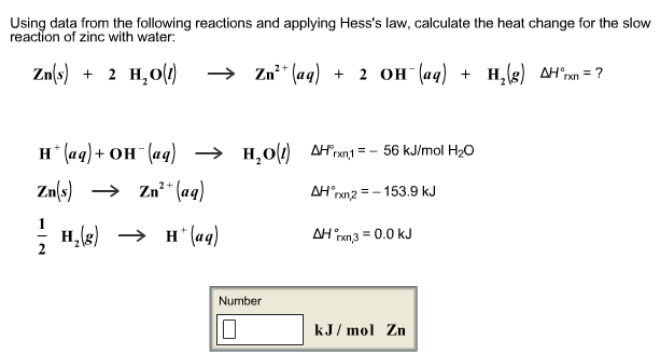# Problem: Using data from the following reactions and applying Hess's law, calculate the heat change for the slow reaction of zinc with water: Zn (s) + 2H2O (l) → Zn2+ (aq) + 2OH- (aq) + H2 (g)    ΔH°rxn = ? H+ (aq) + OH- (aq) → H2O (l)     ΔH°rxn, 1 = -56 kJ/mol H2O Zn (s) → Zn2+ (aq)    ΔH°rxn, 2 = -153.9 kJ 1/2 H2 (g) → H+ (aq)     ΔH°rxn, 3 = 0.0 kJ

###### FREE Expert Solution
97% (206 ratings)###### Problem Details

Using data from the following reactions and applying Hess's law, calculate the heat change for the slow reaction of zinc with water:

Zn (s) + 2H2O (l) → Zn2+ (aq) + 2OH- (aq) + H2 (g)    ΔH°rxn = ?

H+ (aq) + OH- (aq) → H2O (l)     ΔH°rxn, 1 = -56 kJ/mol H2

Zn (s) → Zn2+ (aq)    ΔH°rxn, 2 = -153.9 kJ

1/2 H2 (g) → H+ (aq)     ΔH°rxn, 3 = 0.0 kJ